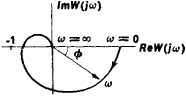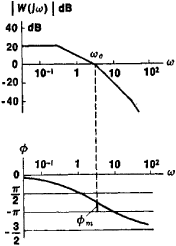Frequency-Response Method

The following article is from The Great Soviet Encyclopedia (1979). It might be outdated or ideologically biased.

Frequency-Response Method

in automatic control theory, a method of evaluating the dynamic characteristics of an automatic control system by using the system’s frequency-response curves expressed as the steady-state response of the system to a harmonic input signal.Figure 1. Amplitude-phase characteristic of an open-loop system

The steady-state response of a stationary linear system to an input signal x1 = A1ejωt is also a harmonic signal x2 = A2ejt + φ). The output and input signals are related by the complex transfer function x2 = W(jω)x1 for which the modulus is given as the ratio of the signal amplitudes | W()| = A2/A1 and the argument W() is given as the phase shift φ(ω) between x2 and x1 The ho-dograph of W() on the complex plane as ω varies from 0 to + ∞ (Figure 1) is called the amplitude-phase characteristic (APC). Every point on the hodograph corresponds to a specific frequency. The length of the vector drawn from the origin of coordinates to a point on the APC corresponding to a frequency ω is equal to | W()|, and the phase shift of the vector relative to the real, positive semiaxis is the argument of W(). The dependence of the modulus and the argument on frequency is expressed by the amplitude-frequency characteristic (AFC) and phase-frequency characteristic (PFC). When the logarithmic amplitude-frequency characteristic (LAFC) and the logarithmic phase-frequency characteristic (LPFC) are plotted, the frequency is laid out along the axis of abscissas on a logarithmic scale, and on the axis of ordinates the values of the modulus expressed in decibels | W() | dB (for LAFC) and of the argument φ(ω) (for LPFC) are laid out on a linear scale (Figure 2). The frequency-response curves are plotted according to the complex transfer function derived from the system’s differential equation or according to the results of measurements of the amplitude ratio and the phase shift between signals at various frequencies. The frequency-response curves (APC or LAFC and LPFC) are used to examine the stability of an automatic control system and the quality indexes of transients in the system. The frequency-response method was introduced into automatic control theory by A. V. Mikhailov between 1936 and 1938.Figure 2. Logarithmic amplitude-frequency and phase-frequency characteristics of an open-loop system

Use of the Nyquist criterion makes it possible to determine beforehand the stability of a closed-loop linear system (that is, a system with feedback) from the APC of the open-loop system; a closed-loop system is stable if the APC of the open-loop system does not enclose the critical point having the coordinates –1, 0 (Figure 1). The stability of the closed-loop system can be evaluated directly from the LAFC and the LPFC of the open-loop system; the closed-loop system is stable if the phase margin φm = π – |φ(ω)c| is positive (Figure 2; ωc is the cutoff frequency at which LAFC intersects the axis of abscissas). The cutoff frequency can be used as a measure of the system’s speed of operation, and the phase margin is useful as a measure of the rate of decay of free oscillations in the system. Logarithmic frequency-response curves and the Nyquist criterion have been used as a basis for developing very effective methods of synthesizing correction devices that ensure the required dynamic characteristics for a closed-loop system. Similar frequency-response methods have been devised for the analysis and synthesis of linear pulse systems. The quality indexes for a transient in a linear system are evaluated with respect to the transient response, which expresses the response of a system to a step signal. The Soviet scientist V. V. Solodovnikov has proposed methods for the synthesis and evaluation of the properties of transient response with respect to the real frequency-response curve P(ω) = ReW(). For nonlinear closed systems the Soviet scientist L. S. Gold’farb has used the frequency-response method to derive a criterion for the occurrence and stability of self-excited oscillations, and the Rumanian mathematician V.-M. Popov has proposed a criterion for absolute stability.

REFERENCES

Voronov, A. A. Osnovy teorii avtomaticheskogo upravleniia, parts 1–2. Moscow, 1965–66.
Teoriia avtomaticheskogo upravleniia, parts 1–2. Moscow, 1968–72.

E. L. L’vov